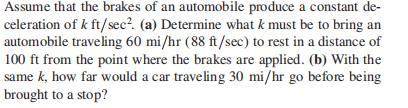### Still have math questions?Assume that the brakes of an automobile produce a constant de- celeration of $$k ft / sec ^ { 2 }$$ . (a) Determine what $$k$$ must be to bring an automobile traveling $$60 mi / hr ( 88 ft / sec )$$ to rest in a distance of $$100 ft$$ from the point where the brakes are applied. (b) With the same $$k$$ , how far would a car traveling $$30 mi / hr$$ go before being brought to a stop?
$$\left. \begin{array} { l l } { \text { (a) } k = - 38.72 } & { \text { (b) } 25 ft } \end{array} \right.$$